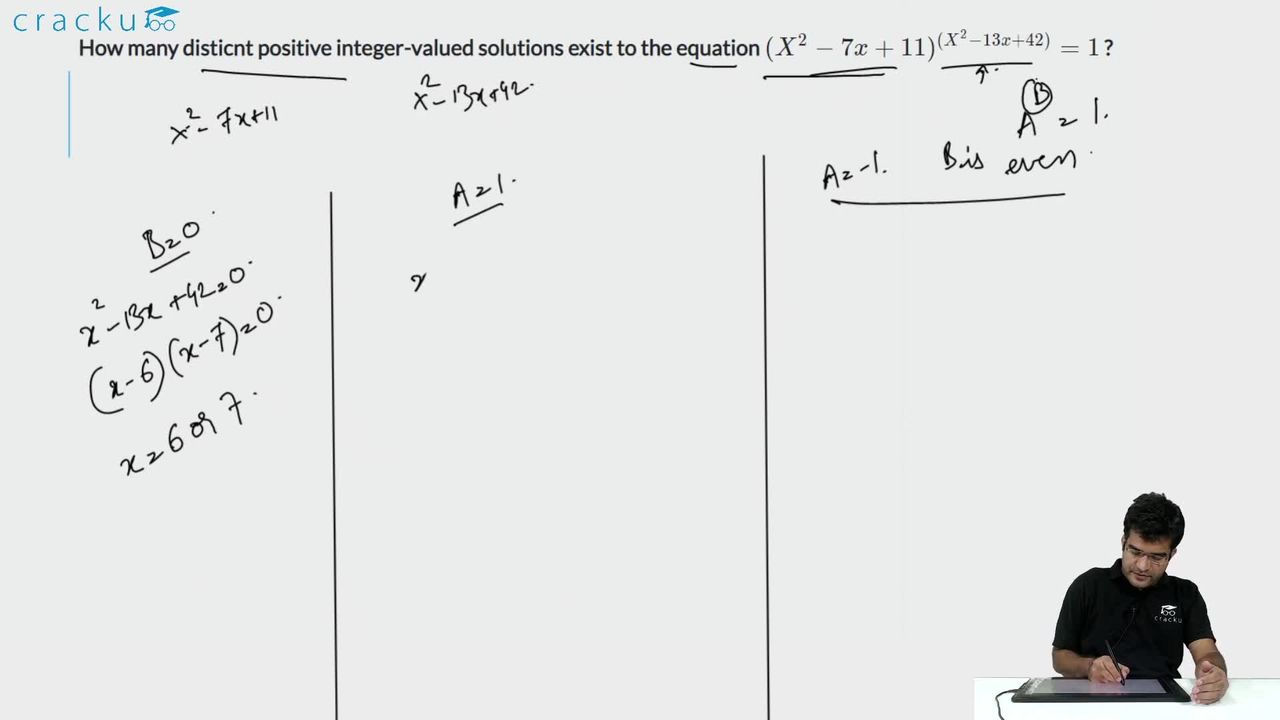Question 55

# How many disticnt positive integer-valued solutions exist to the equation $$(x^{2}-7x+11)^{(x^{2}-13x+42)}=1$$ ?

Solution

$$(x^{2}-7x+11)^{(x^{2}-13x+42)}=1$$

if $$(x^{2}-13x+42)$$=0 or $$(x^{2}-7x+11)$$=1 or $$(x^{2}-7x+11)$$=-1 and $$(x^{2}-13x+42)$$ is even number

For x=6,7 the value $$(x^{2}-13x+42)$$=0

$$(x^{2}-7x+11)$$=1 for x=5,2.

$$(x^{2}-7x+11)$$=-1 for x=3,4 and for X=3 or 4, $$(x^{2}-13x+42)$$ is even number.

.'. {2,3,4,5,6,7} is the solution set of x.

.'. x can take six values.

### View Video Solution